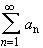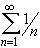#Interactive Real Analysis

Next | Previous | Glossary | Map

## 4.1. Series and Convergence

### Theorem: Absolute Convergence implies Convergence

If a seriesconverges absolutely, it converges in the ordinary sense. The converse is not true.

Supposeis absolutely convergent. Let

Tj = |a1| + |a2| + ... + |aj|

be the sequence of partial sums of absolute values, and

Sj = a1 + a2 + ... + aj

be the "regular" sequence of partial sums. Since the series converges absolutely, there exists an integer N such that:

| Tn - Tm| = |an| + |an-1| + ... + |am+2| + |am+1| <if n > m > N. But we have by the triangle inequality that

| Sn - Sm| = | an + an-1 + ... + am+2 + am+1 ||an| + |an-1| + ... + |am+2| + |am+1| = | Tn - Tm | <Hence the sequence of regular partial sums {Sn} is Cauchy and therefore must converge (compare this proof with the Cauchy Criterion for Series).

The converse is not true because the seriesconverges, but the corresponding series of absolute valuesdoes not converge.

Next | Previous | Glossary | Map